Скачать презентацию Introduction to computer storing instructions and information

3c5db3c32a21ea807489989363a96f58.ppt

• Количество слайдов: 29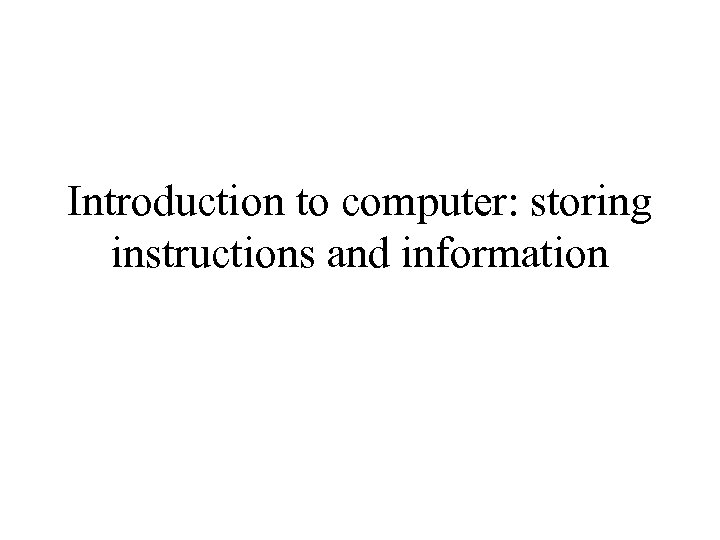Introduction to computer: storing instructions and information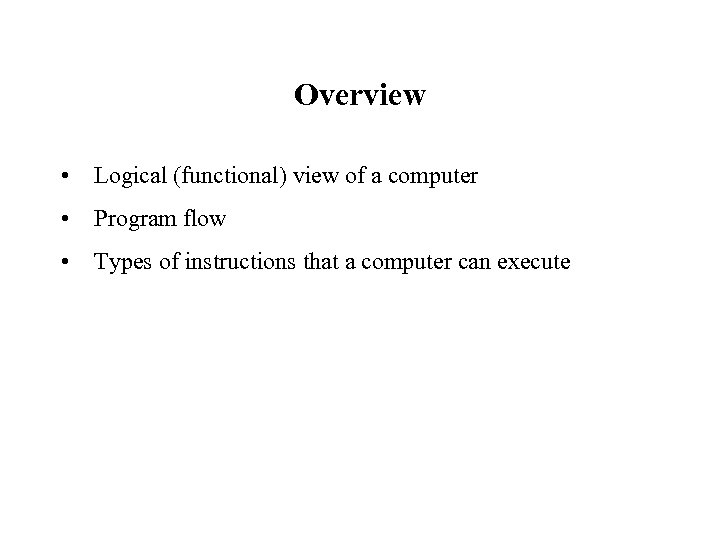Overview • Logical (functional) view of a computer • Program flow • Types of instructions that a computer can execute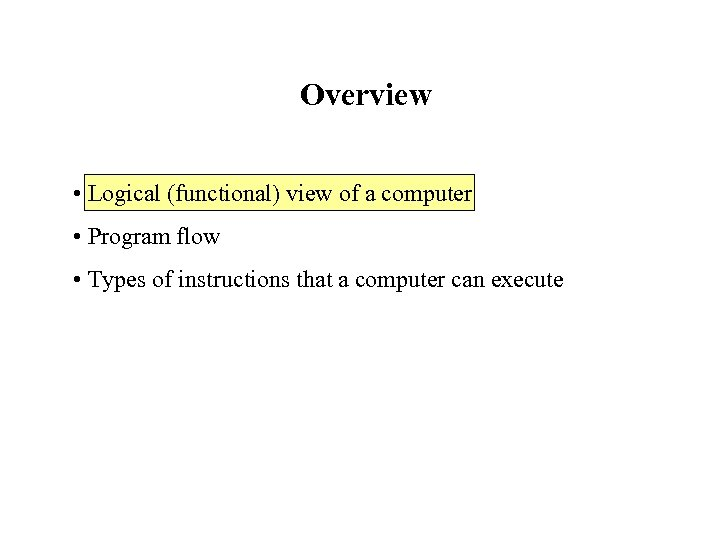Overview • Logical (functional) view of a computer • Program flow • Types of instructions that a computer can execute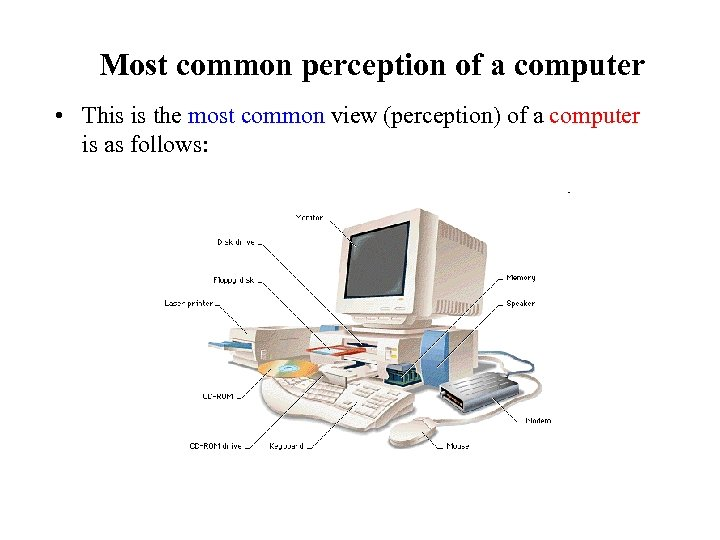Most common perception of a computer • This is the most common view (perception) of a computer is as follows: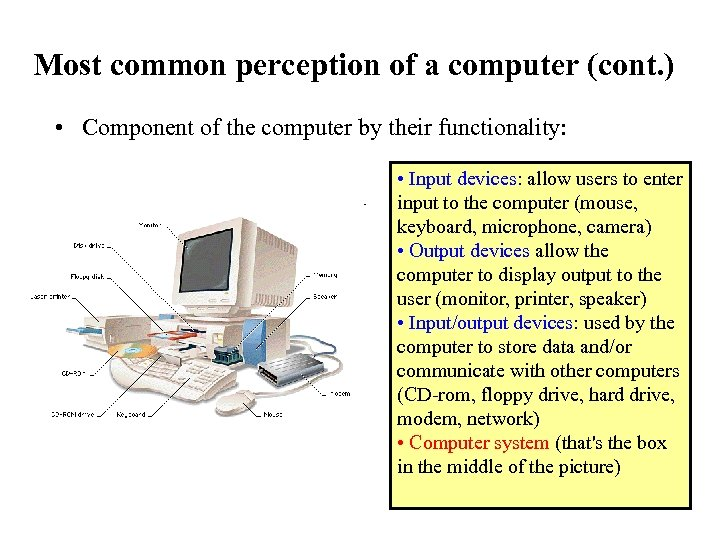Most common perception of a computer (cont. ) • Component of the computer by their functionality: • Input devices: allow users to enter input to the computer (mouse, keyboard, microphone, camera) • Output devices allow the computer to display output to the user (monitor, printer, speaker) • Input/output devices: used by the computer to store data and/or communicate with other computers (CD-rom, floppy drive, hard drive, modem, network) • Computer system (that's the box in the middle of the picture)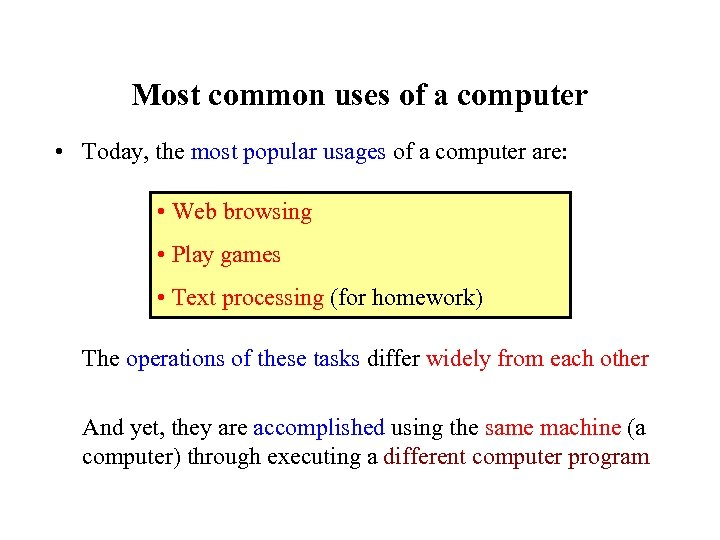Most common uses of a computer • Today, the most popular usages of a computer are: • Web browsing • Play games • Text processing (for homework) The operations of these tasks differ widely from each other And yet, they are accomplished using the same machine (a computer) through executing a different computer program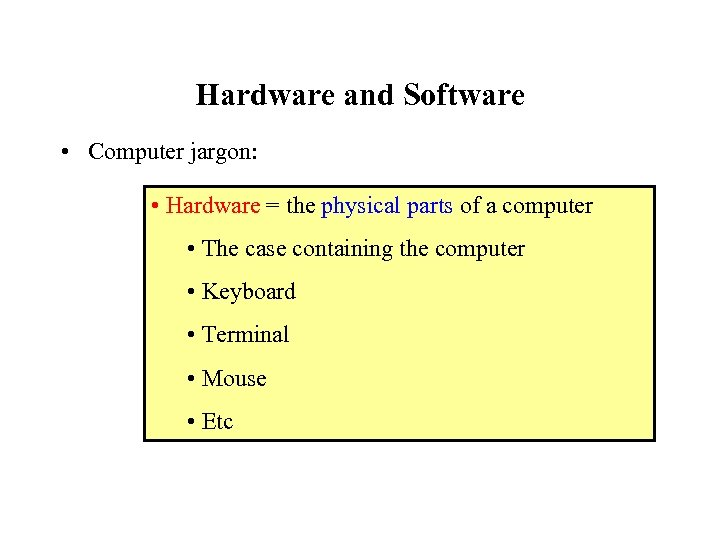Hardware and Software • Computer jargon: • Hardware = the physical parts of a computer • The case containing the computer • Keyboard • Terminal • Mouse • Etc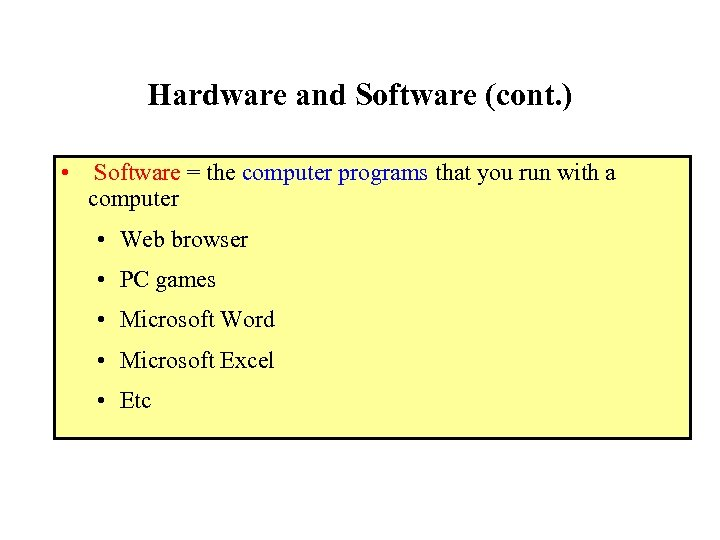Hardware and Software (cont. ) • Software = the computer programs that you run with a computer • Web browser • PC games • Microsoft Word • Microsoft Excel • Etc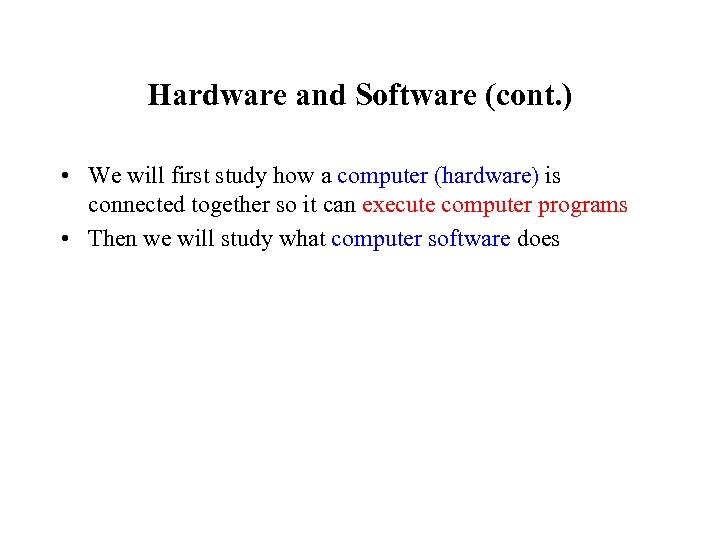Hardware and Software (cont. ) • We will first study how a computer (hardware) is connected together so it can execute computer programs • Then we will study what computer software does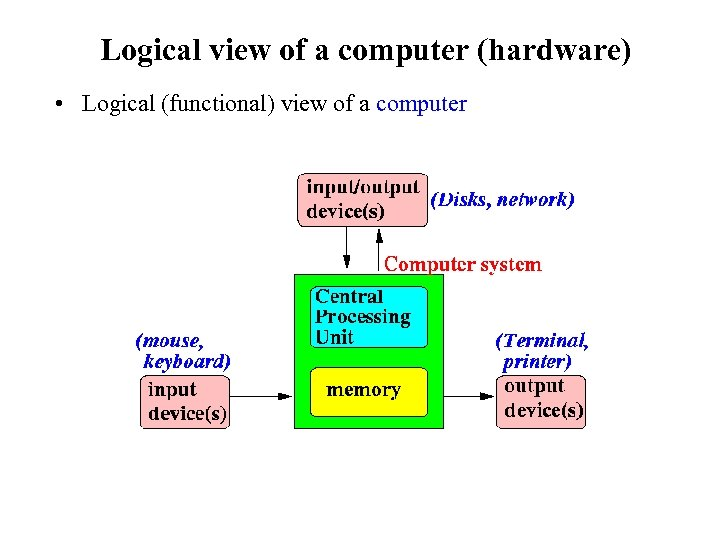Logical view of a computer (hardware) • Logical (functional) view of a computer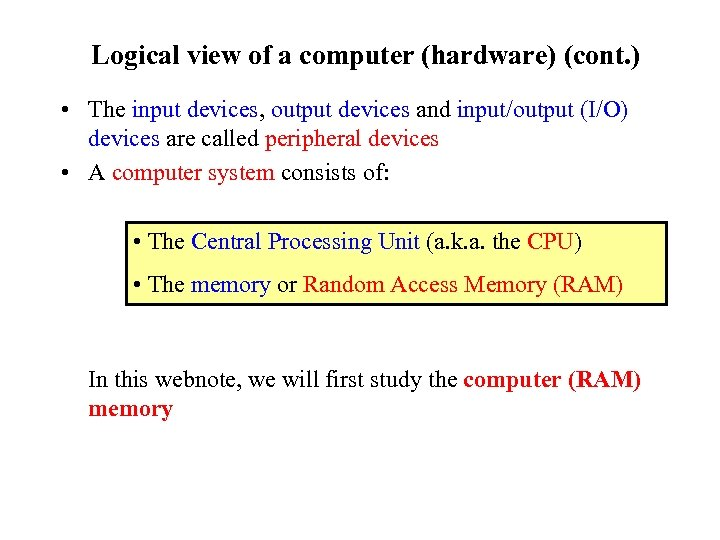Logical view of a computer (hardware) (cont. ) • The input devices, output devices and input/output (I/O) devices are called peripheral devices • A computer system consists of: • The Central Processing Unit (a. k. a. the CPU) • The memory or Random Access Memory (RAM) In this webnote, we will first study the computer (RAM) memory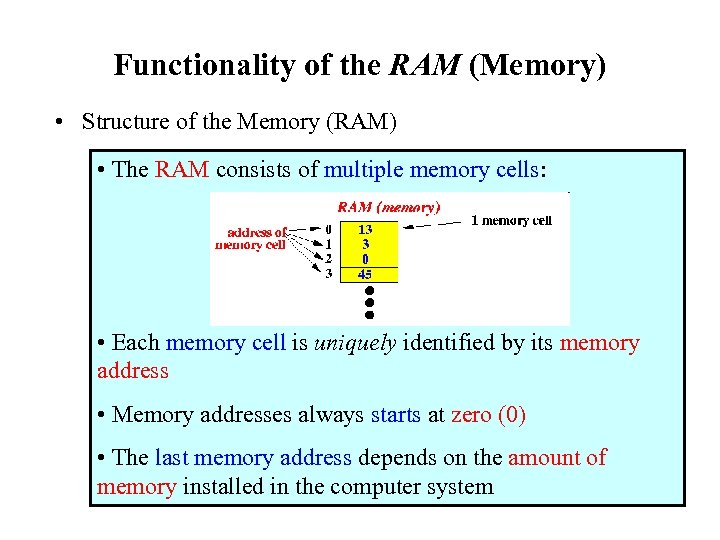Functionality of the RAM (Memory) • Structure of the Memory (RAM) • The RAM consists of multiple memory cells: • Each memory cell is uniquely identified by its memory address • Memory addresses always starts at zero (0) • The last memory address depends on the amount of memory installed in the computer system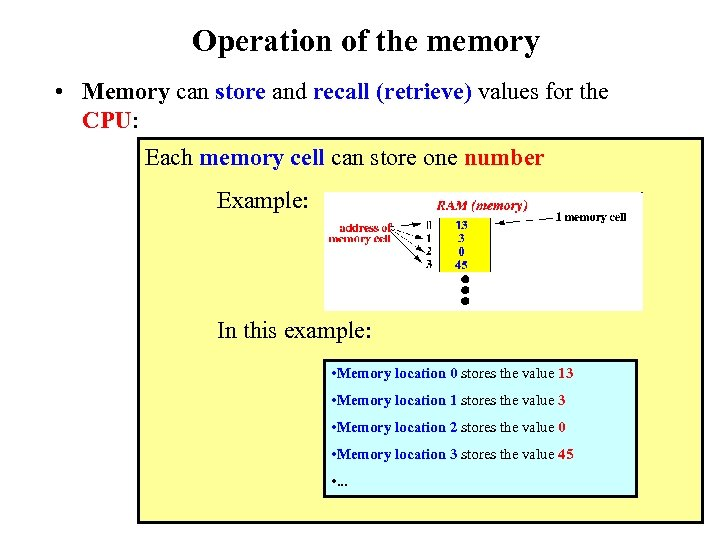Operation of the memory • Memory can store and recall (retrieve) values for the CPU: Each memory cell can store one number Example: In this example: • Memory location 0 stores the value 13 • Memory location 1 stores the value 3 • Memory location 2 stores the value 0 • Memory location 3 stores the value 45 • . . .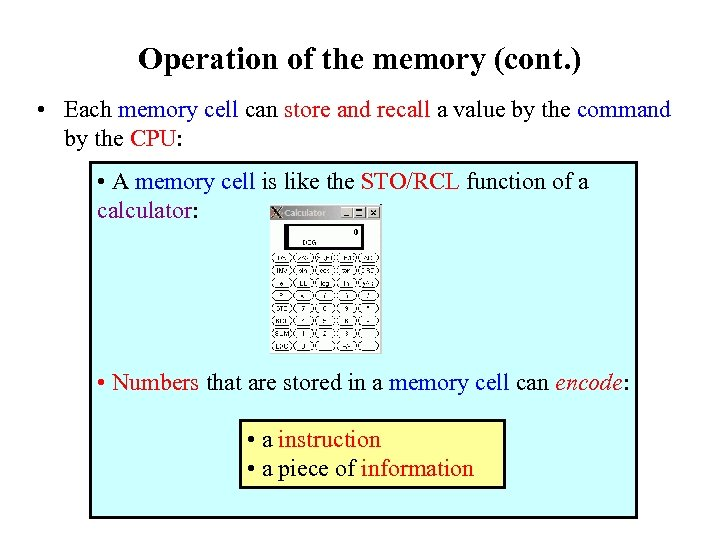Operation of the memory (cont. ) • Each memory cell can store and recall a value by the command by the CPU: • A memory cell is like the STO/RCL function of a calculator: • Numbers that are stored in a memory cell can encode: • a instruction • a piece of information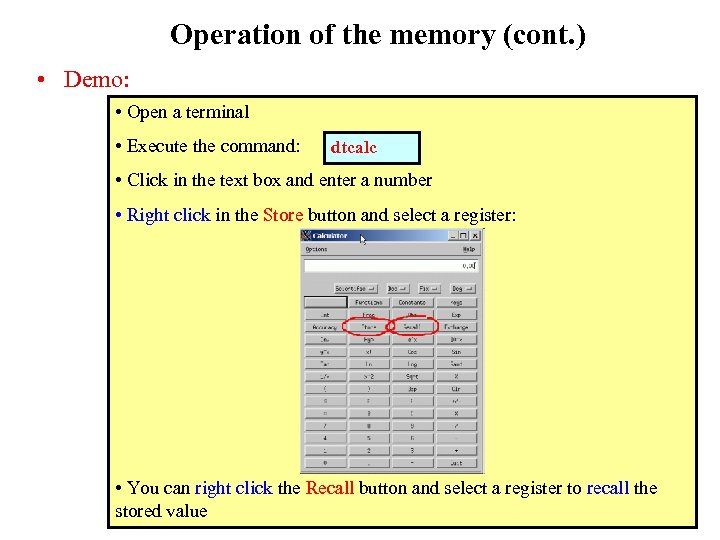Operation of the memory (cont. ) • Demo: • Open a terminal • Execute the command: dtcalc • Click in the text box and enter a number • Right click in the Store button and select a register: • You can right click the Recall button and select a register to recall the stored value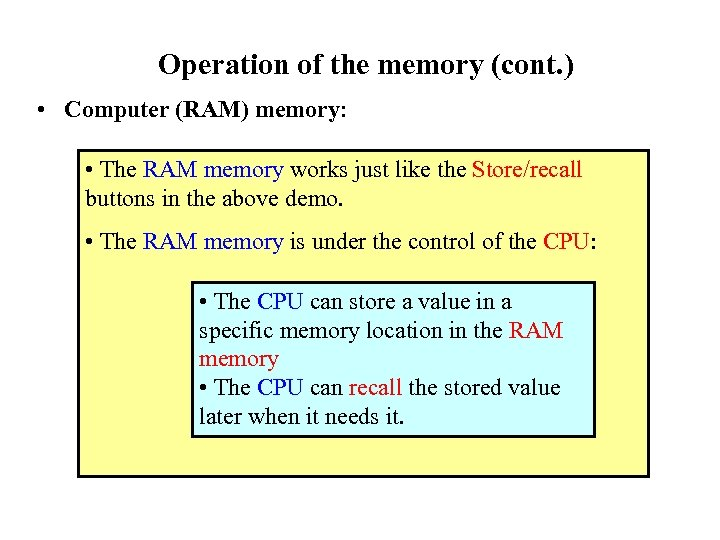Operation of the memory (cont. ) • Computer (RAM) memory: • The RAM memory works just like the Store/recall buttons in the above demo. • The RAM memory is under the control of the CPU: • The CPU can store a value in a specific memory location in the RAM memory • The CPU can recall the stored value later when it needs it.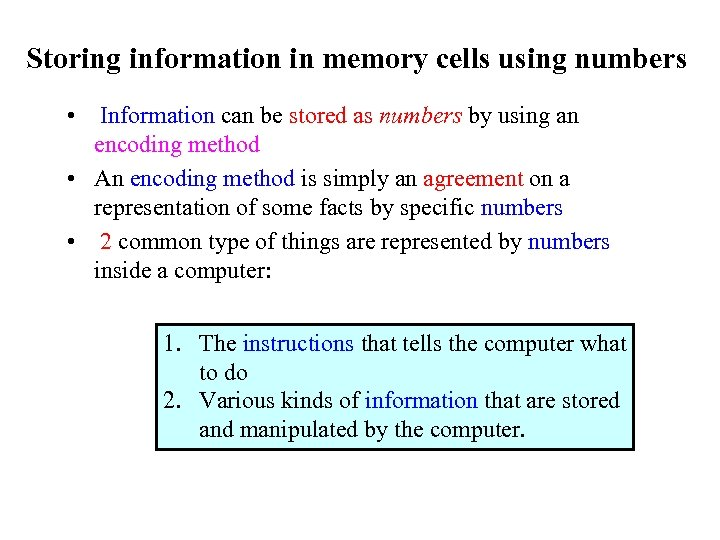Storing information in memory cells using numbers • Information can be stored as numbers by using an encoding method • An encoding method is simply an agreement on a representation of some facts by specific numbers • 2 common type of things are represented by numbers inside a computer: 1. The instructions that tells the computer what to do 2. Various kinds of information that are stored and manipulated by the computer.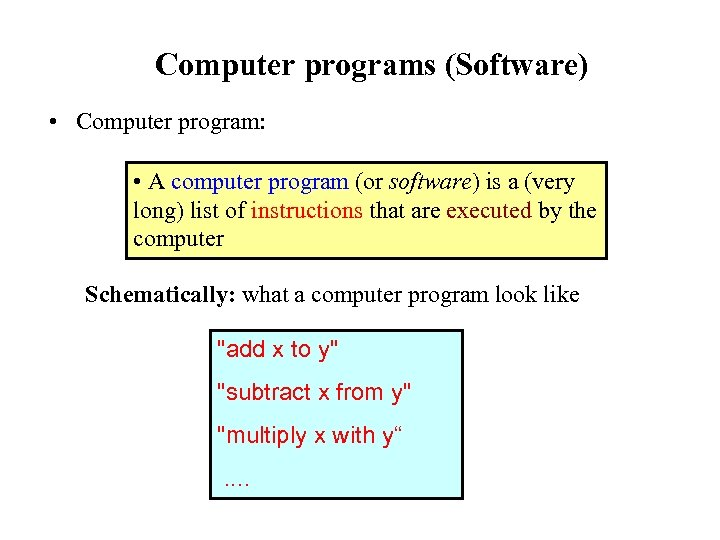Computer programs (Software) • Computer program: • A computer program (or software) is a (very long) list of instructions that are executed by the computer Schematically: what a computer program look like "add x to y" "subtract x from y" "multiply x with y“. .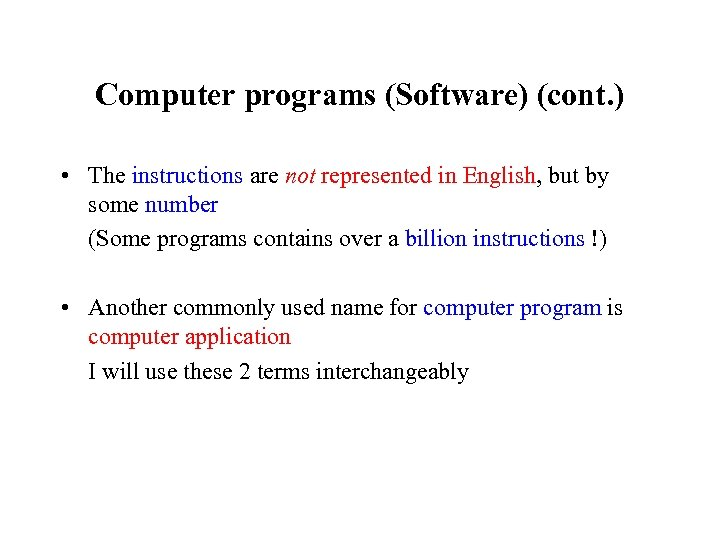Computer programs (Software) (cont. ) • The instructions are not represented in English, but by some number (Some programs contains over a billion instructions !) • Another commonly used name for computer program is computer application I will use these 2 terms interchangeably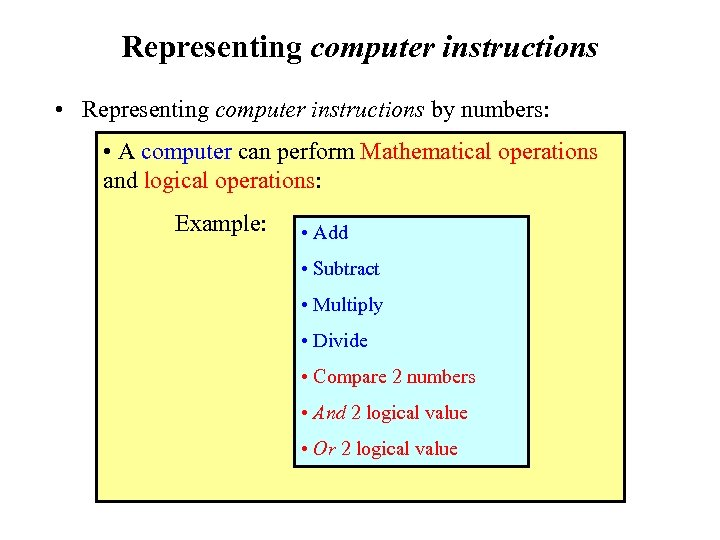Representing computer instructions • Representing computer instructions by numbers: • A computer can perform Mathematical operations and logical operations: Example: • Add • Subtract • Multiply • Divide • Compare 2 numbers • And 2 logical value • Or 2 logical value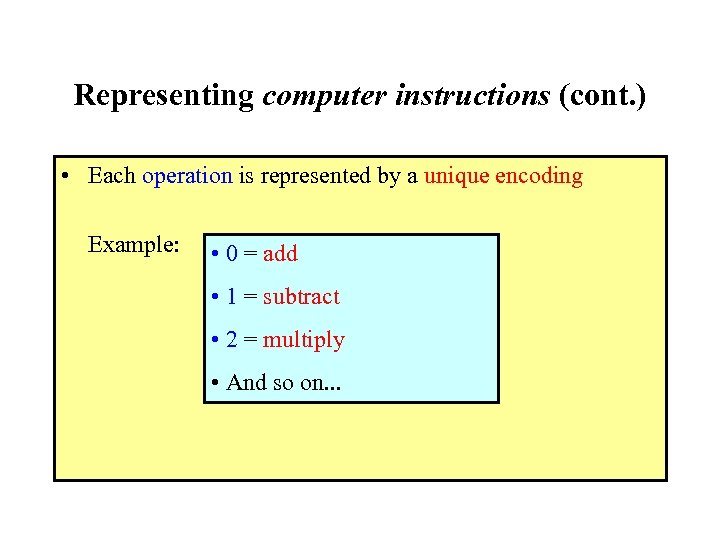Representing computer instructions (cont. ) • Each operation is represented by a unique encoding Example: • 0 = add • 1 = subtract • 2 = multiply • And so on. . .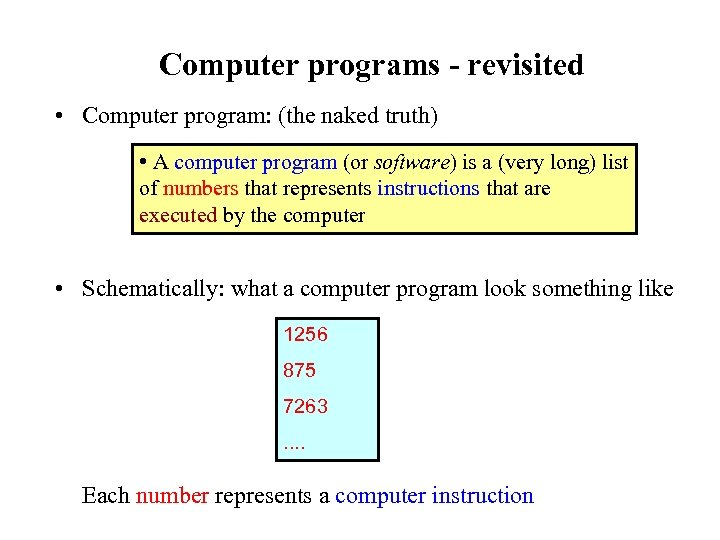Computer programs - revisited • Computer program: (the naked truth) • A computer program (or software) is a (very long) list of numbers that represents instructions that are executed by the computer • Schematically: what a computer program look something like 1256 875 7263. . Each number represents a computer instruction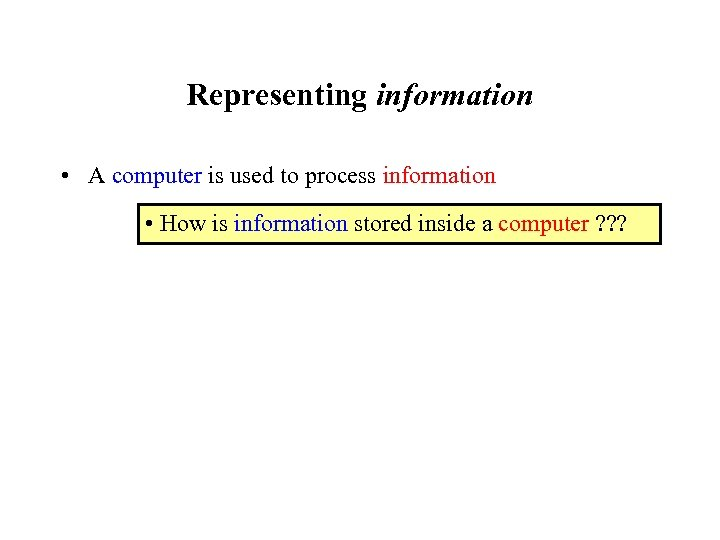Representing information • A computer is used to process information • How is information stored inside a computer ? ? ?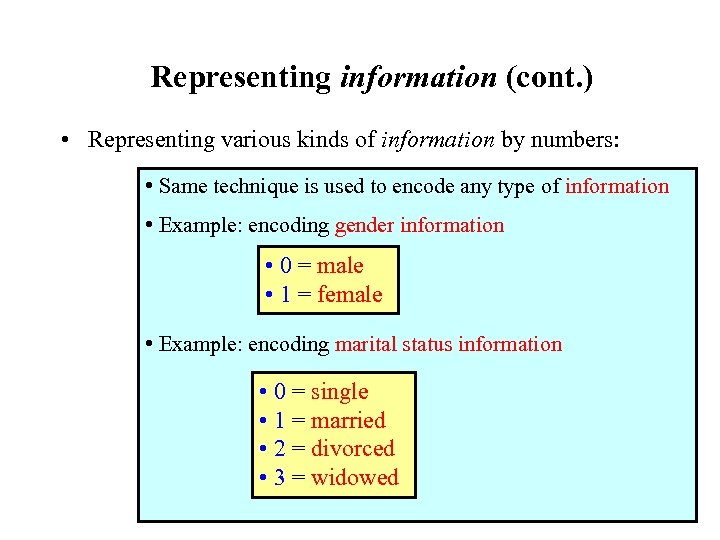Representing information (cont. ) • Representing various kinds of information by numbers: • Same technique is used to encode any type of information • Example: encoding gender information • 0 = male • 1 = female • Example: encoding marital status information • 0 = single • 1 = married • 2 = divorced • 3 = widowed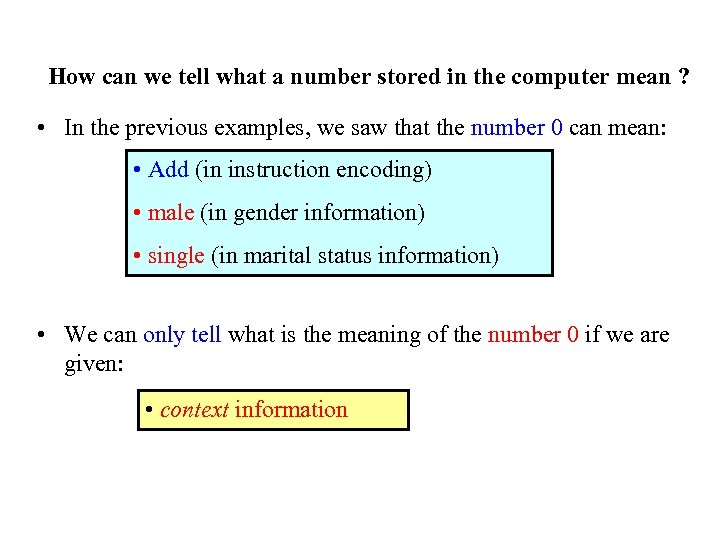How can we tell what a number stored in the computer mean ? • In the previous examples, we saw that the number 0 can mean: • Add (in instruction encoding) • male (in gender information) • single (in marital status information) • We can only tell what is the meaning of the number 0 if we are given: • context information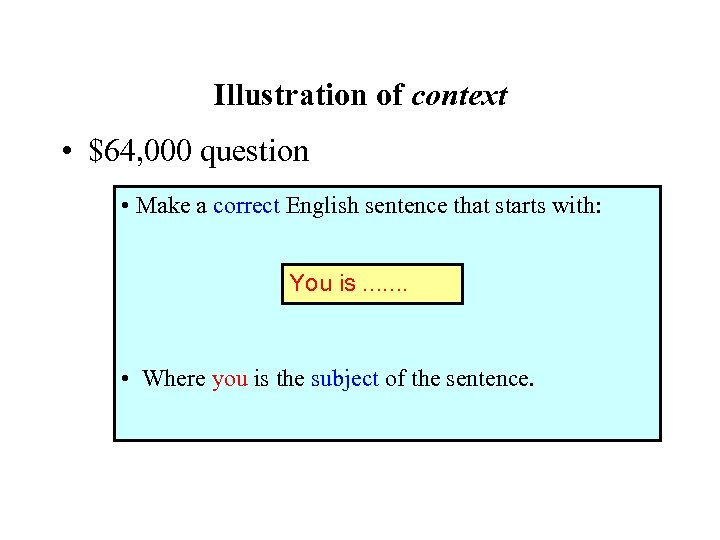Illustration of context • \$64, 000 question • Make a correct English sentence that starts with: You is. . . . • Where you is the subject of the sentence.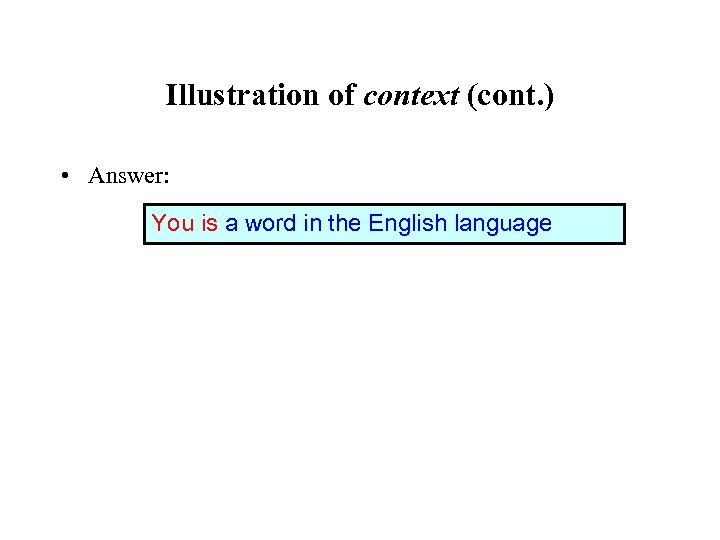Illustration of context (cont. ) • Answer: You is a word in the English language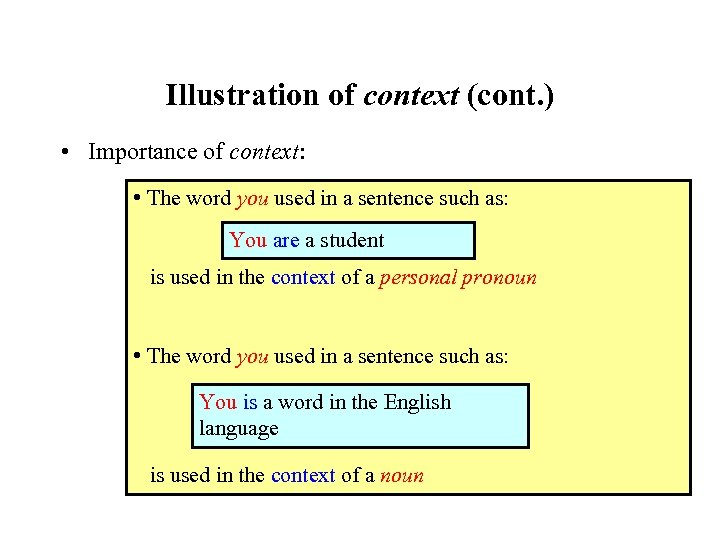Illustration of context (cont. ) • Importance of context: • The word you used in a sentence such as: You are a student is used in the context of a personal pronoun • The word you used in a sentence such as: You is a word in the English language is used in the context of a noun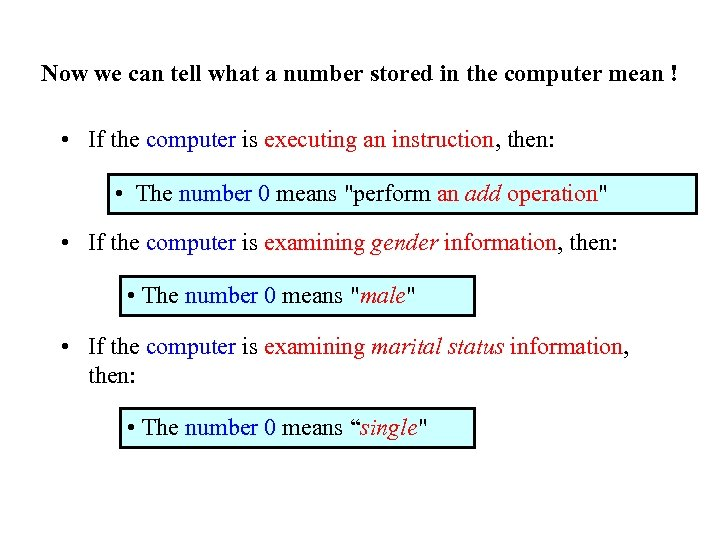Now we can tell what a number stored in the computer mean ! • If the computer is executing an instruction, then: • The number 0 means "perform an add operation" • If the computer is examining gender information, then: • The number 0 means "male" • If the computer is examining marital status information, then: • The number 0 means “single"